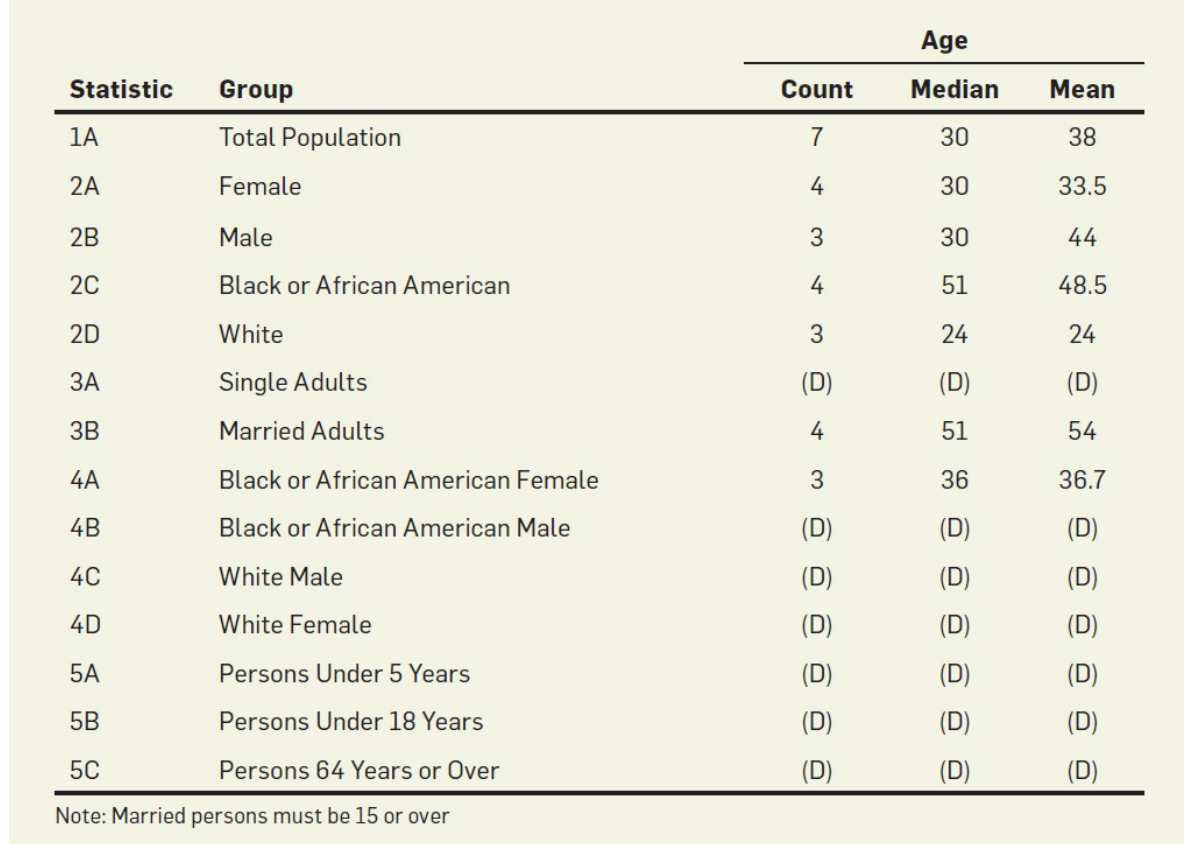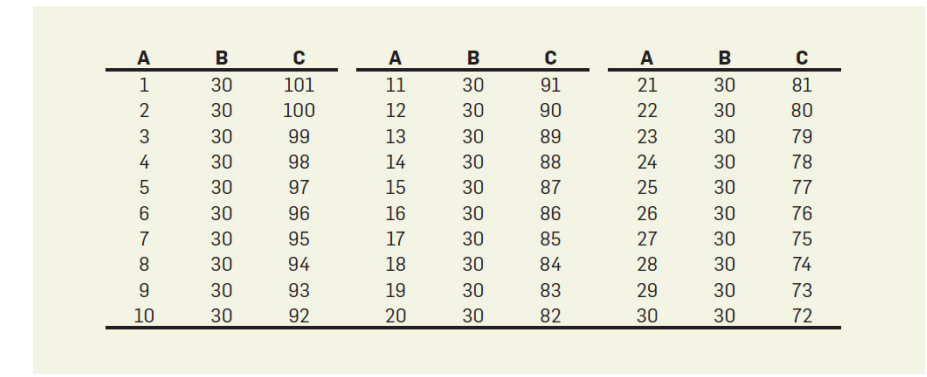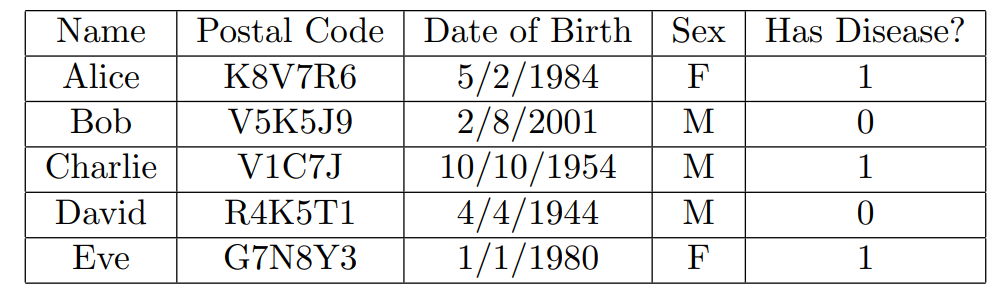# L2-Reconstruction Attacks

## 裂解聚合（Cracking Aggregation）• 首先注意到表中有4位黑人，三位女黑人，为此可以推断出有一位男黑人
• 然后再通过$$2B$$行通过排列组合以及中位数和平均数推断出三位公民的年龄大致处于### 总结反思

• 虽然发动聚合裂解攻击都是一个$$NP$$难问题，但是通过人工启发式的做法就能排除掉大部分的无效数据
• 同理这种攻击在美国2010年人口普查中足以重构出$$46%$$的人口的小数据，同时对于$$71$$的人口，其误差不大于一年。通过一些附加的商业数据集，可以重新辨别出$$50$$万人的姓名

## 查询（Queries），全然非隐私（BNP）以及Dinur-Nissim攻击

• 给定一个带有几个数据（points）的列表
• 首先定义每一个数据为行（rows）
• 定义特征或者说每条数据的维度为列（columns）
• 有一些特征为唯一标识符，例如说名字，邮政编码以及出生年月日和性别等，这里的标识符都是完全公开的
• 其中一个二进制位特征作为使用的隐私符号
• 其中$$d\in \{0,1\}^n$$为隐私符的向量### 模型架构

$A(S) = d \cdot S$

$r(S)=A(S)$

### 总结

• 第一种攻击需要$$2^n$$次的查询以消除$$O(n)$$的噪音
• 第二种攻击需要$$\Omega(n)$$次的查询以消除$$O(\sqrt n)$$的噪音（还有加强版本）

posted @ 2021-03-07 21:05  Uzuki  阅读(1109)  评论(0编辑  收藏  举报